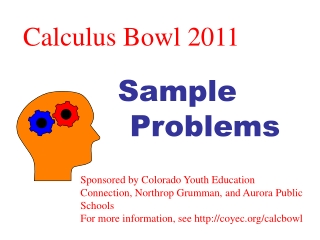DownloadDownload PresentationCalculus Bowl 2011

# Calculus Bowl 2011

Download Presentation## Calculus Bowl 2011

- - - - - - - - - - - - - - - - - - - - - - - - - - - E N D - - - - - - - - - - - - - - - - - - - - - - - - - - -
##### Presentation Transcript

1. Calculus Bowl 2011 Sample Problems Sponsored by Colorado Youth Education Connection, Northrop Grumman, and Aurora Public Schools For more information, see http://coyec.org/calcbowl

2. Problem 1 (d) Derivative of constant = 0

3. Problem 2 (d)

4. Problem 3 (a)

5. Problem 4 4cos(πx-2)+1=4cos[π(x+P)-2]+1 P=2

6. Problem 5 (c)

7. Problem 6 (d)

8. Problem 7 (d) Second derivative

9. Problem 8 (b) y = ln x

10. Problem 9 (e)

11. Problem 10 P(x) (2,2) (-1, -1) q(-1) = p(-2) = 0

12. Problem 11 When did you have \$3000 in your account? Or When did you earn \$2000 in interest? (d)

13. Problem 12 (c)

14. Problem 13 y y=p(x) (c)

15. Problem 14 g(x) (2,1) (e)

16. Problem 15

17. Problem 16 2 cos(0.25θ) = 2 cos[0.25(θ+P)] (d)

18. Problem 17 (c)

19. Problem 19 (a)

20. Problem 20

21. Problem 21

22. Problem 22 y x

23. Problem 23

24. Problem 25

25. Problem 26

26. Problem 28

27. Problem 29

28. Problem 31